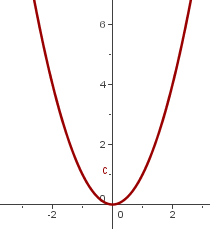Learn from home

The teachersGraph y = x²

xy = x²
-24
-11
00
11
24## 1. Vertical Translation

y = x² + k

If k > 0, y = x² moves upward k units.

If k < 0, y = x² moves down k units.

The vertex of the parabola is: (0, k).

The axis of symmetry is: x = 0.y = x² + 2 y = x² − 2

## 2. Horizontal Translation

y = (x + h)²

If h > 0, y = x² is shifted to the left h units.

If h < 0, y = x² is shifted to the right h units.

The vertex of the parabola is: (−h, 0).

The axis of symmetry is: x = −h.y = (x + 2)²y = (x − 2)²

## 3. Oblique Translation

y = (x + h)² + k

The vertex of the parabola is: (−h, k).

The axis of symmetry is: x = −h.y = (x − 2)² + 2 y = (x + 2)² − 2

Did you like the article?(1 votes, average: 5.00 out of 5)Loading...

Emma

I am passionate about travelling and currently live and work in Paris. I like to spend my time reading, gardening, running, learning languages and exploring new places.

Did you like
this resource?

Bravo!